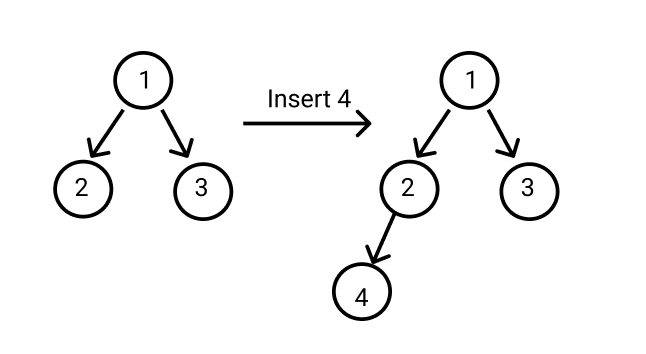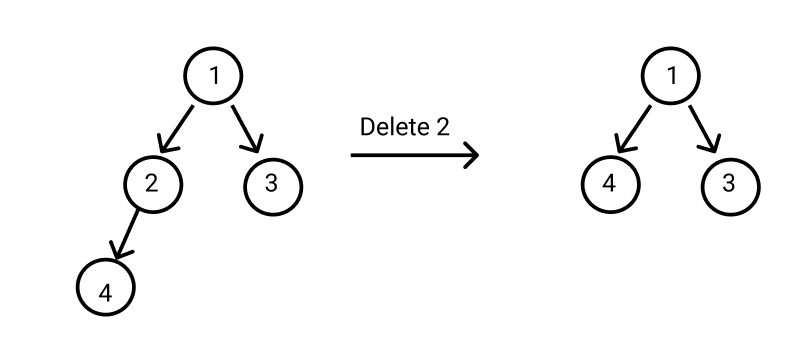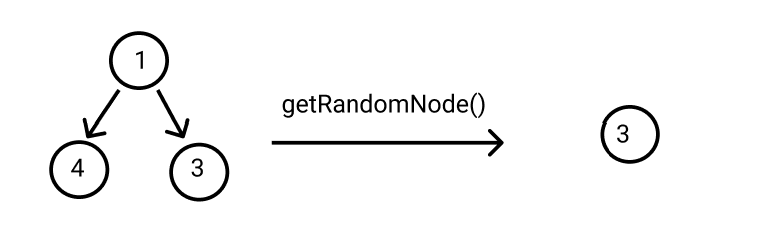New update is available. Click here to update.

# Ninja and Binary Tree

Last Updated: 7 Mar, 2021
Difficulty: Moderate

## PROBLEM STATEMENT

#### For example:##### Input Format
``````The first line of input contains an integer 'T' representing the number of test cases. Then the test cases follow.

The next line of each test case contains an integer ‘Q’ representing the number of queries to Ninja.

The next line, ‘Q’ lines of each test case, contain a character and integer representing which type of query is to perform on the binary tree and the value of the node.
``````
##### Output Format :
``````For each test case, return the value of the Random Node obtained from the get Random Node query.

Print the output of each test case in a separate line.
``````

#### Note:

``````You do not need to print anything; it has already been taken care of. Just implement the given function.
``````
##### Constraints :
``````1 <= ‘T’ <= 10
1 <= ‘Q’ <= 10000
Char = {‘I’, ‘D’, ‘R’}
1 <= ‘VAL’ <= 100000

Time Limit: 1 second
``````## Approach 1

In this problem, we have to implement a binary tree class from scratch. We have to deal with 3 queries: Insert a node in the binary tree, Delete a  node in the binary tree and get a random node in the binary tree. So we make three functions to deal with these three queries.

For inserting a node in the binary tree, first, we do iterative level order traversal in the given binary tree using a queue. If we find a node whose left/right child is empty, we make a new node with ‘VAL’ as the data of the node and add this node into the left/right child in the binary tree.

For deleting a node in the binary tree, first, we start from the root of the binary tree then find the deepest and rightmost node of the binary tree and the node which we want to delete. Then we replace the deepest rightmost node value with the node to be deleted. Then we delete the deepest right node.

For finding a random node in the binary tree, first, we store the inorder traversal of the binary tree in an array/list. Then we generate a random number between 0 to a number of nodes - 1 and return the value which is present at that random index in the array/list.

Here is the algorithm :

Insertion in the binary tree :

1. If the root of the binary tree is null:
• Return a new node with the value ‘VAL’.
2. We declare a queue in which we store nodes of the binary tree.
3. Add the root of the binary tree in the queue.
4. We run a loop while the queue is not empty:
• Remove the first element from the queue.
• If the left node of the current node is ‘NULL’ :
• Add a new node with the value ‘VAL’ to the left of the current node.
• Return root.
• If the right node of this node is ‘NULL’ :
• Add a new node with the value ‘VAL’ to the right of the current node.
• Return root.
• Add left and right nodes of the current node into the queue.

Deletion in the binary tree :

1. If the root node is ‘NULL’:
• Return.
2. If we are at the leaf node of the binary tree:
• Return.
3. We declare a queue in which we store nodes of the binary tree.
4. Add the root of the binary tree in the queue.
5. We declare a node ‘NODE_TO_BE_DELETED’  and ‘DEEPEST_NODE’ in which we store a node that we have to delete and the rightmost deepest node in the binary tree.
6. We run a loop while the queue is not empty:
• Remove the first element from the queue and store into ‘DEEPEST_NODE’
• If the ‘DEEPEST_NODE’ value is equal to ‘VAL’:
• ‘NODE_TO_BE_DELETED’ = ‘DEEPEST_NODE’
• If the left node of the ‘DEEPEST_NODE’ is not ‘NULL’:
• Add the left node of the ‘DEEPEST_NODE’ into the queue.
• If the right node of the ‘DEEPEST_NODE’ is not ‘NULL’:
• Add right node of the ‘DEEPEST_NODE’ into the queue
7. If ‘NODE_TO_BE_DELETED’ is not ‘NULL’:
• ‘DEEPEST_NODE_VAL’ = ‘DEEPEST_NODE.DATA’
• DELETE_DEEPEST_NODE( ROOT,‘DEEPEST_NODE’).
• ‘NODE_TO_BE_DELETED.DATA’ = ‘DEEPEST_NODE_VAL’.

Function DELETE_DEEPEST_NODE( ROOT,‘DEEPEST_NODE’):

1. We traverse on the binary tree using level order traversal.
2. If the node of the binary tree is ‘DEEPEST_NODE’:
• Remove this node.

Get random node from the binary tree:

1. We declare an array/list ‘ALL_NODES’ in which we store all nodes of the binary tree.
2. We traverse on the binary tree and store all the nodes in ‘ALL_NODES’.
3. We generate a  random number between 0 to a number of nodes - 1.
• Return the value which is present at that random index.